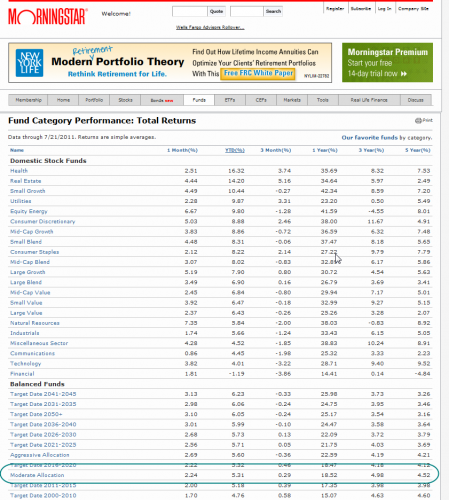Note: This blog was  updated for additional information in January 2022.

In 2009 when visiting North Carolina, we stayed with dear friends on Lake Norman. After a day of boating I asked our friend what the large signs sticking out of the tops of telephone poles in the middle of the lake were, he said they marked the shoals. Without these signs, boaters unfamiliar with the location of shoals could beach their crafts. The concept of shoals in regards boating can also be applied when analyzing your fiduciary account.

There are shoals that we need to watch out for in our fiduciary practices that may not be well posted; it is up to us to protect our clients. Sometimes fiduciaries are unsure whether or not their accounts are doing well or doing poorly. The reason for the uncertainty is twofold:

1. Performance is difficult to calculate
2. Performance is relative and they do not know what to compare their accounts’ performance to

Here is a quick and easy way to calculate approximate performance. Let’s presume this is a fiduciary account that has a separate working capital account that normally covers the expenses, so the investment account has had no deposits and no withdrawals. The “return” formula is: divide the ending value by the beginning value and subtract one (1) as shown under “Example #1”  below.

### Examples of Calculating Return on Investment

Example #1: The account grew from \$100,000 to \$120,000.

120,000 / 100,000 = 1.2. Subtract 1 and you get 0.2, or 20%. If that happened over, say 16 months, multiply the 20% by 12/16 (the number of months in a year divided by the number of months in the actual period). In this example, 20% x 12  /16 = 15% per year.

Now let’s suppose that there were withdrawals (distributions from the account). The formula is: ending value + withdrawal, divided by beginning value, minus one. So supposing you use the above example and add withdrawals of \$4,000 you will see the results in Example #2 (below).

Example #2: The account grew from \$100,000 to \$120,000 and there were withdrawals of \$4,000.

(120,000 + 4,000) / 100,000 = 1.24.  Subtract 1, and you get 0.24 or 24%. Example #3 below shows how to calculate a return with both withdrawals and deposits.

Example #3: The account grew from \$100,000 to \$120,000, there were withdrawals of \$4,000 and a deposit of \$10,000.

(120,000 + 4,000 – 10,000) / 100,000 = 1.14. Subtract 1 and you get 0.14, or 14%. Example #4 shows how to calculate a return if you started with \$100,000, but ended up with less, say \$90,000, after also receiving \$4,000 in distributions.

Example #4: The account dropped from \$100,000 to \$90,000 and there were withdrawals of \$4,000.

(90,000 + 4,000)/  100,000 = 0.94. Subtract 1, and you get -0.06 or -6% return (a 6% loss).

### Online Resources to Determine Performance

Now that you have the performance, you want to know if it is good or bad for a fiduciary account. Since the Morningstar Moderate Allocation Category of funds represents a set of diversified mutual funds, it would probably comply with the Prudent Investor Rule and, therefore, provides a good comparison. Here is access to the Moderate Allocation Fund average.Scroll down and you will see the Balanced Funds heading and underneath it Moderate Allocation. Look at the 1-year performance to compare with the performance you have calculated above (the periods must be the same, however, to make a proper comparison – just a month or two difference can invalidate the comparison).If you will regularly review your fiduciary account using the rough methodology above, you will begin to fulfill your fiduciary obligation to monitor. This will also give you a rough idea about risk and help you avoid the shoals in your fiduciary practice. If the performance is significantly below the benchmark over the most recent year, then you have probably taken much higher risk.